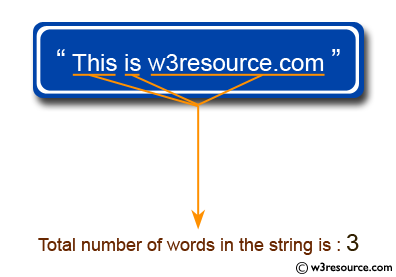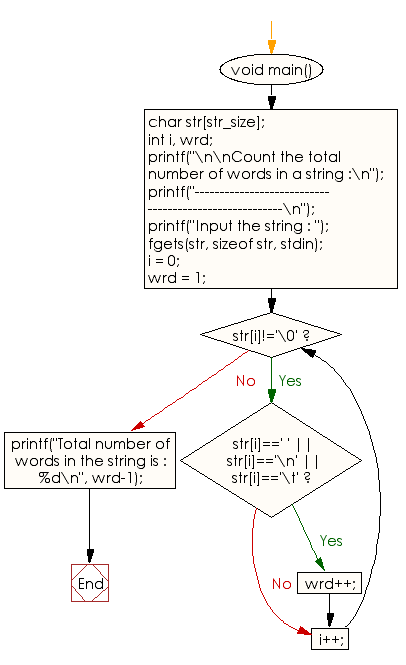﻿ C exercises: Count the total number of words in a string - w3resource# C Programming: Count the total number of words in a string

## C String: Exercise-5 with Solution

Write a program in C to count the total number of words in a string.Sample Solution:

C Code:

``````#include <stdio.h>
#include <string.h>
#include <stdlib.h>

#define str_size 100 //Declare the maximum size of the string

void main()
{
char str[str_size];
int i, wrd;

printf("\n\nCount the total number of words in a string :\n");
printf("------------------------------------------------------\n");
printf("Input the string : ");
fgets(str, sizeof str, stdin);

i = 0;
wrd = 1;

/* loop till end of string */
while(str[i]!='\0')
{
/* check whether the current character is white space or new line or tab character*/
if(str[i]==' ' || str[i]=='\n' || str[i]=='\t')
{
wrd++;
}

i++;
}

printf("Total number of words in the string is : %d\n", wrd-1);
}
```
```

Sample Output:

```Count the total number of words in a string :
------------------------------------------------------
Input the string : This is w3resource.com
Total number of words in the string is : 3
```

Flowchart:C Programming Code Editor:

Improve this sample solution and post your code through Disqus.

What is the difficulty level of this exercise?

﻿